The GLM Procedure

### Construction of Least Squares Means

To construct a least squares mean (LS-mean) for a given level of a given effect, construct a row vector
according to the following rules and use it in an ESTIMATE statement to compute the value of the LS-mean:

1. Set all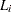corresponding to covariates (continuous variables) to their mean value.

2. Consider effects contained by the given effect. Set thecorresponding to levels associated with the given level equal to 1. Set all otherin these effects equal to 0. (See Chapter 15, The Four Types of Estimable Functions, for a definition of containing.)

3. Consider the given effect. Set thecorresponding to the given level equal to 1. Set thecorresponding to other levels equal to 0.

4. Consider the effects that contain the given effect. If these effects are not nested within the given effect, then set thecorresponding to the given level to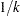, whereis the number of such columns. If these effects are nested within the given effect, then set thecorresponding to the given level to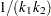, where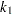is the number of nested levels within this combination of nested effects, and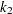is the number of such combinations. Forcorresponding to other levels, use 0.

5. Consider the other effects not yet considered. If there are no nested factors, then set allcorresponding to this effect to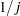, whereis the number of levels in the effect. If there are nested factors, then set allcorresponding to this effect to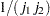, where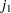is the number of nested levels within a given combination of nested effects and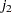is the number of such combinations.

The consequence of these rules is that the sum of the Xs within any classification effect is 1. This set of Xs forms a linear combination of the parameters that is checked for estimability before it is evaluated.

For example, consider the following model:

```proc glm;
class A B C;
model Y=A B A*B C Z;
lsmeans A B A*B C;
run;
```

Assume A has 3 levels, B has 2 levels, and C has 2 levels, and assume that every combination of levels of A and B exists in the data. Assume also that Z is a continuous variable with an average of 12.5. Then the least squares means are computed by the following linear combinations of the parameter estimates:

 A B A*B C1 2 3 1 2 11 12 21 22 31 32 1 2 Z LSM( ) 1 1/3 1/3 1/3 1/2 1/2 1/6 1/6 1/6 1/6 1/6 1/6 1/2 1/2 12.5 LSM(A1) 1 1 0 0 1/2 1/2 1/2 1/2 0 0 0 0 1/2 1/2 12.5 LSM(A2) 1 0 1 0 1/2 1/2 0 0 1/2 1/2 0 0 1/2 1/2 12.5 LSM(A3) 1 0 0 1 1/2 1/2 0 0 0 0 1/2 1/2 1/2 1/2 12.5 LSM(B1) 1 1/3 1/3 1/3 1 0 1/3 0 1/3 0 1/3 0 1/2 1/2 12.5 LSM(B2) 1 1/3 1/3 1/3 0 1 0 1/3 0 1/3 0 1/3 1/2 1/2 12.5 LSM(AB11) 1 1 0 0 1 0 1 0 0 0 0 0 1/2 1/2 12.5 LSM(AB12) 1 1 0 0 0 1 0 1 0 0 0 0 1/2 1/2 12.5 LSM(AB21) 1 0 1 0 1 0 0 0 1 0 0 0 1/2 1/2 12.5 LSM(AB22) 1 0 1 0 0 1 0 0 0 1 0 0 1/2 1/2 12.5 LSM(AB31) 1 0 0 1 1 0 0 0 0 0 1 0 1/2 1/2 12.5 LSM(AB32) 1 0 0 1 0 1 0 0 0 0 0 1 1/2 1/2 12.5 LSM(C1) 1 1/3 1/3 1/3 1/2 1/2 1/6 1/6 1/6 1/6 1/6 1/6 1 0 12.5 LSM(C2) 1 1/3 1/3 1/3 1/2 1/2 1/6 1/6 1/6 1/6 1/6 1/6 0 1 12.5

#### Setting Covariate Values

By default, all covariate effects are set equal to their mean values for computation of standard LS-means. The AT option in the LSMEANS statement enables you to set the covariates to whatever values you consider interesting.

If there is an effect containing two or more covariates, the AT option sets the effect equal to the product of the individual means rather than the mean of the product (as with standard LS-means calculations). The AT MEANS option leaves covariates equal to their mean values (as with standard LS-means) and incorporates this adjustment to crossproducts of covariates.

As an example, the following is a model with a classification variable A and two continuous variables, x1 and x2:

```class A;
model y = A x1 x2 x1*x2;
```

The coefficients for the continuous effects with various AT specifications are shown in the following table.

 Syntax x1 x2 x1*x2 lsmeans A;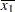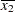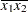lsmeans A / at means;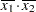lsmeans A / at x1=1.2; 1.2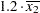lsmeans A / at (x1 x2)=(1.2 0.3); 1.2 0.3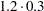For the first two LSMEANS statements, the A LS-mean coefficient for x1 is(the mean of x1) and for x2 is(the mean of x2). However, for the first LSMEANS statement, the coefficient for x1*x2 is, but for the second LSMEANS statement the coefficient is. The third LSMEANS statement sets the coefficient for x1 equal to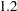and leaves that for x2 at, and the final LSMEANS statement sets these values toand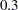, respectively.

Even if you specify a WEIGHT variable, the unweighted covariate means are used for the covariate coefficients if there is no AT specification. However, if you also use an AT specification, then weighted covariate means are used for the covariate coefficients for which no explicit AT values are given, or if you specify AT MEANS. Also, observations with missing dependent variables are included in computing the covariate means, unless these observations form a missing cell. You can use the E option in conjunction with the AT option to check that the modified LS-means coefficients are the ones you want.

The AT option is disabled if you specify the BYLEVEL option, in which case the coefficients for the covariates are set equal to their means within each level of the LS-mean effect in question.

#### Changing the Weighting Scheme

The standard LS-means have equal coefficients across classification effects; however, the OM option in the LSMEANS statement changes these coefficients to be proportional to those found in the input data set. This adjustment is reasonable when you want your inferences to apply to a population that is not necessarily balanced but has the margins observed in the original data set.

In computing the observed margins, PROC GLM uses all observations for which there are no missing independent variables, including those for which there are missing dependent variables. Also, if there is a WEIGHT variable, PROC GLM uses weighted margins to construct the LS-means coefficients. If the analysis data set is balanced or if you specify a simple one-way model, the LS-means will be unchanged by the OM option.

The BYLEVEL option modifies the observed-margins LS-means. Instead of computing the margins across the entire data set, PROC GLM computes separate margins for each level of the LS-mean effect in question. The resulting LS-means are actually equal to raw means in this case. The BYLEVEL option disables the AT option if it is specified.

Note that the MIXED procedure implements a more versatile form of the OM option, enabling you to specifying an alternative data set over which to compute observed margins. If you use the BYLEVEL option, too, then this data set is effectively the "population" over which the population marginal means are computed. See Chapter 56, The MIXED Procedure, for more information.

You might want to use the E option in conjunction with either the OM or BYLEVEL option to check that the modified LS-means coefficients are the ones you want. It is possible that the modified LS-means are not estimable when the standard ones are, or vice versa.Previous Page | Next Page | Top of Page